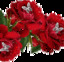Trending ▼   ResFinder# CBSE Pre Board Class 10 2020 : Mathematics

5 pages, 48 questions, 0 questions with responses, 0 total responses,30Manickavalli S  Coimbatore +Fave Message ProfileTimelineUploads
 Home > valliammu >   F Also featured on: cbse10_pre_boards cbse10Formatting page ...

Practice Paper X Class I Subject: Mathematics (041) Time:3 Hours Maximum Marks: 80 l. All 2. Please write down the Serial Number of the question before attempting it. 3' the questions are compulsory. The question paper consists of 40 questions and it is divided into four sections A, B, Section A comprises of 20 questions c and D. carrying I mark each. 5. Section B comprises of 6 questions carrying 2 marks each. 6. Section C comprises of g questions carrying 3 marks each. 7. Section D comprises of 6 questions carrying 4 marks each. 8' There is no overall choice' However, an internal choice has been provided in two questions of I mark' two questions of 2 marks, three questions of 3 marks and three questions of 4 marks each. you have to attempt onry one ofthe alternatives in a, such questions. 9. Use of calculator is not permitted. 4 ) Section 1. If HCF of (26, 1691:13, then LCM (26,169) (A)26 (B) -A is s2 (c) 33s If cos A + cos2A: l, then the value of sin2A * riroa i, (A)-l 3. (B)0 (c)l . 13 @)2 L45a7 The decimal expansion of the rational nunlber (A)One decimalplace (B) Two decimal places (D) will terminate after l;;- (C) Three decimal places (D)Four decimalplaces 4. The distance between the points (A) 6 5. units (B) The pair ofequation 5x 8 units - l5y (A)One solution (B) Two solutions A (0, 6) and B (0, _ 2) is (C) 4 = g and 3x _ 9y : f units (D) 2 units nu, (C) Infinite solutions (D)No solutions 6. The area of a triangle with vertices (3, 0), (7, 0) and (g, 4) is (A) 14 sq' units (B) 2g sq. units (c) 8 sq. units (D) 6 sq. units 7 ' A tower stands vertically on the ground. From a poini o, the ground which is 25 m awayfrom the foot of the tower, the angle of elevation of the top of the tower is found to be 450. Then the height (in meters) of the tower is 8' (^) 2slT (g) 25y'3 G)2s rtP(:,4) is the mid-point of the line segmentjoining the points value of a is (A)-8 (B)3 (c)_4 (D) l2.s A (- 6, 5) and B (-2,3), then the @)4

Related ResPapers
 CBSE Pre Board Class 10 2021 : Mathematics (New Horizon Public School (NHPS), Panvel) by gladisj CBSE Class 10 Pre Board 2021 : Mathematics Standard - Set 5 (CBSE Gulf Sahodaya Qatar Chapter, Doha) by cbse10_pre_boards CBSE Class 10 Pre Board 2020 : Mathematics Basic - Prelim 1 (Kendriya Vidyalaya (KV) Agra) by cbse10_pre_boards CBSE Pre Board Class 10 2020 : Mathematics (Gokhale Memorial Girls School, Kolkata) by sumanta4evrFormatting page ...Formatting page ...Formatting page ...Formatting page ...